# Slope of a Line

#### Topics

• Slope of a Line Or Gradient of a Line.
• Parallelism of Line
• Perpendicularity of Line in Term of Slope
• Collinearity of Points
• Slope of a line when coordinates of any two points on the line are given
• Conditions for parallelism and perpendicularity of lines in terms of their slopes
• Angle between two lines
• Collinearity of three points

## Definition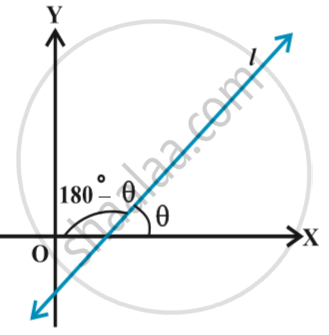If θ is the inclination of a line l, then tan θ is called the slope or gradient of the line l. The slope of a line whose inclination is 90° is not defined. The slope of a line is denoted by m. Thus, m = tan θ, θ ≠ 90°
It may be observed that the slope of x-axis is zero and slope of y-axis is not defined.

## Notes

Slope of a line when coordinates of any two points on the line are given:
To find the slope of a line in terms of the coordinates of two points on the line.
Let P(x_1, y_1) and Q(x_2, y_2) be two points on non-vertical line l whose inclination is θ. Obviously, x_1 ≠ x_2, otherwise the line will become perpendicular to x-axis and its slope will not be defined. The inclination of the line l may be acute or obtuse. Let us take these two cases.
Draw perpendicular QR to x-axis and PM perpendicular to RQ as shown in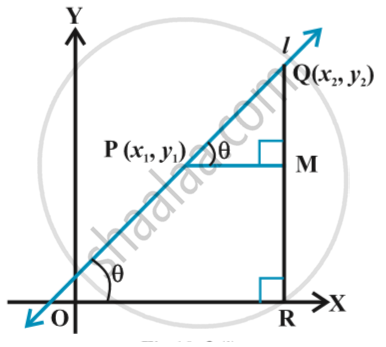Case 1 :  When angle  θ is acute:
∠MPQ =  θ      . ... (1)
Therefore, slope of line l = m = tan θ.
But in triangle MPQ, we have tan theta = (MQ)/(MP) = (y_2 -y_1)/(x_2 - x_1)    ....(2)
From equations (1) and (2), we have
m = (y_2-y_1)/(x_2 -x_1)

Case 2: When angle θ is obtuse: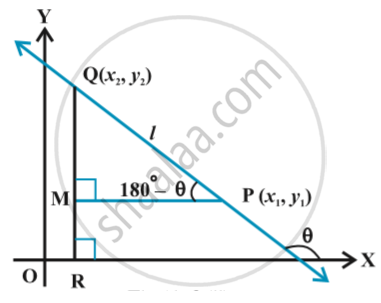we have ∠MPQ = 180° –  θ.
Therefore,  θ = 180° – ∠MPQ.
Now, slope of the line l
m = tan θ
= tan ( 180° – ∠MPQ) = – tan  ∠MPQ
=(-(MQ)/(MP)) = (-y_2-y_1)/(x_1-x_2) =(y_2-y_1)/(x_2-x_1)
Consequently, we see that in both the cases the slope m of the line through the points
(x_1, y_1) and (x_2, y_2) is given by m =(y_2-y_1)/(x_2-x_1).

Conditions for parallelism and perpendicularity of lines in terms of their slopes:
In a coordinate plane, suppose that non-vertical lines l_1 and l_2 have slopes m_1 and m_2, respectively. Let their inclinations be α and β, respectively. If the line l_1 is parallel to l_2.
then their inclinations are equal, i.e.,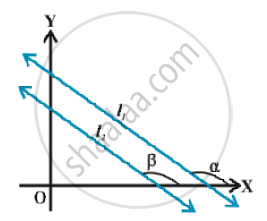α = β, and hence, tan α = tan β
Therefore m_1 = m_2, i.e., their slopes are equal.
Conversely, if the slope of two lines l_1 and l_2 is same, i.e.,
m_1 = m_2  Then tan α = tan β.
By the property of tangent function (between 0° and 180°), α = β. Therefore, the lines are parallel.
Hence, two non vertical lines l_1 and l_2 are parallel if and only if their slopes are equal.

Angle between two lines :
Let L_1 and L_2 be two non-vertical lines with slopes m_1 and m_2, respectively. If α_1 and α_2 are the inclinations of lines L_1 and L_2, respectively. Then
m_1 = tanα_1 and m_2 = tan α_2.
two lines intersect each other, they make two pairs of vertically opposite angles such that sum of any two adjacent angles is 180°. Let θ and φ be the adjacent angles between the lines L_1 and L_2. Then  θ = α_2 – α_1 and α_1, α_2  ≠ 90°.
Therefore  tan θ = tan (α_2 – α_1) = (tanα_2 - tanα_1)/( 1+tanα_1tanα _2) = (m_2-m_1)/(1+m_1m_2)       (as 1 + m1m2 ≠ 0)
and φ = 180° – θ so that
tan φ = tan (180° – θ ) = – tan θ = -(m_2-m_1)/(1+m_1m_2) , as 1 + m1m2 ≠ 0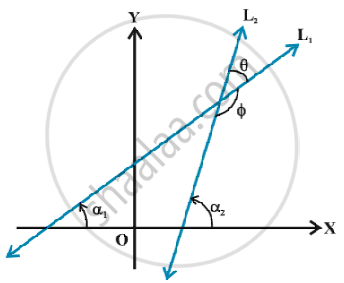Collinearity of three points:
We know that slopes of two parallel lines are equal. If two lines having the same slope pass through a common point, then two lines will coincide. Hence, if A, B and C are three points in the XY-plane, then they will lie on a line, i.e., three points are collinear.  if and only if slope of AB = slope of BC.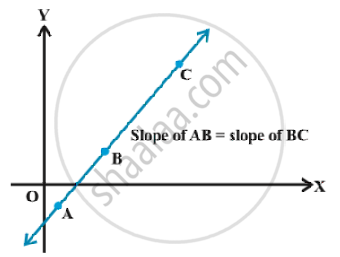If you would like to contribute notes or other learning material, please submit them using the button below.

### Shaalaa.com

Angle between two lines [00:12:35]
S
0%